FanDuel World Series Odds
+400
4 to 1Yankees
17.1% implied probability

+450
4.5 to 1Dodgers
15.6% implied probability

+600
6 to 1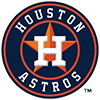Astros
12.2% implied probability

+700
7 to 1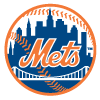Mets
10.7% implied probability

+1300
13 to 1Padres
6.1% implied probability

+1300
13 to 1Blue Jays
6.1% implied probability

+1300
13 to 1Braves
6.1% implied probability

+1800
18 to 1Brewers
4.5% implied probability

+2800
28 to 1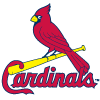Cardinals
3.0% implied probability

+3000
30 to 1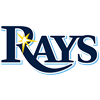Rays
2.8% implied probability

+3000
30 to 1Giants
2.8% implied probability

+3200
32 to 1Red Sox
2.6% implied probability

+3500
35 to 1White Sox
2.4% implied probability

+4000
40 to 1Twins
2.1% implied probability

+4500
45 to 1Phillies
1.9% implied probability

+7500
75 to 1Angels
1.1% implied probability

+7500
75 to 1Guardians
1.1% implied probability

+12000
120 to 1Mariners
0.7% implied probability

+20000
200 to 1Marlins
0.4% implied probability

+30000
300 to 1Rangers
0.3% implied probability

+100000
1000 to 1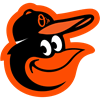Orioles
0.1% implied probability

+250000
2500 to 1Diamondbacks
0.0% implied probability

+250000
2500 to 1Royals
0.0% implied probability

+250000
2500 to 1Tigers
0.0% implied probability

+250000
2500 to 1Rockies
0.0% implied probability

+500000
5000 to 1Pirates
0.0% implied probability

+500000
5000 to 1Athletics
0.0% implied probability

+500000
5000 to 1Reds
0.0% implied probability

+500000
5000 to 1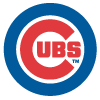Cubs
0.0% implied probability

+500000
5000 to 1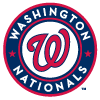Nationals
0.0% implied probability